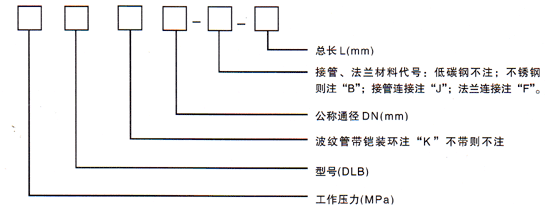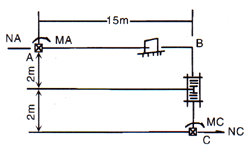﻿大拉杆补偿器|横向补偿器-大拉杆横向波纹补偿器(TDL)-中创机械

首页 | 收藏 | 地图

连接方式：1、法兰连接 2、接管连接 补偿量：根据用户要求二、大拉杆补偿器横向补偿器）使用说明：
可以补偿弯曲管段的横向位移和角位移。
三、大拉杆横补偿器对支座作用力的计算：

L-管架到横向补偿器中点的距离　　 m。
四、大拉杆补偿器应用举例（不考虑温度对补偿量及刚度的修正）Y=160mm，查样本可选用0.6DLB500-FB-2000，横向补偿量Y0=193mm，横向刚度Ky=22N/mm
（2）A、C支座受力计算

FA=Ky·160/2=22×80=1760(N)　　 MA=FA×2=1760×2=3520（N·m）
FB=Ky·160/2=22×80=1760(N)　　 MB=FB ×2=1760×2=3520（N·m）